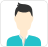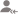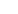Find the best tutors and institutes for Class 12 Tuition

Find Best Class 12 Tuition

No matching category found.

No matching Locality found.

Outside India?

Search for topicsPost a Lesson

All

All

Lessons

Discussion

Lesson Posted on 08 Jan CBSE/Class 11/Science/Chemistry/Unit 1-Basic Concepts of ChemistryShijo Francis

I have qualified central govt. Exams for fellowship to pursue ph.D in cusat. So I have good idea in...

Organic chemistry means how particular reagents will react. Example if we considering acid it will make a proton donor or an electron acceptor. That is when was an acid is added it would acidify the medium by donating the proton. Similarly base will add hydroxyl up. But it some exceptional cases are... read more

Organic chemistry means how particular reagents will react. Example if we considering acid it will make a proton donor or an electron acceptor. That is when was an acid is added it would acidify the medium by donating the proton. Similarly base will add hydroxyl up. But it some exceptional cases are there where it would not react. That is it will act as the medium for the reaction to take place — nitration reaction sulphuric acid act as the medium.

Dislike Bookmark

Answered on 27/11/2018 CBSE/Class 11/Science/Chemistry/Unit 1-Basic Concepts of Chemistry

How is empirical formula of a compound related to its molecular formula?Anany

Molecular formula = empirical formula * n-factor ( where n-factor = molecular mass / empirical mass )
Dislike Bookmark

Answered on 28/10/2018 CBSE/Class 11/Science/Chemistry CBSE/Class 11/Science/Chemistry/Unit 1-Basic Concepts of ChemistryPapiya Abedin

Chemistry Expert

The S I unit of mass is kg.
Dislike Bookmark

Looking for Class 12 Tuition

Find best Class 12 Tuition in your locality on UrbanPro.

FIND NOW

Answered on 15 Jan CBSE/Class 11/Science/Chemistry CBSE/Class 11/Science/Chemistry/Unit 1-Basic Concepts of ChemistrySufiyan Chauhan

Chemistry Tutor in Bathinda

"0.50 mol Na2CO3" means 0.50 x 106(molar mass) =53g of solid Na2CO3 ,whereas "0.50 M Na2CO3" means that 0.50 mol of Na2CO3 (53g) is present in 1 litre of solution.
Dislike Bookmark

Answered on 05/11/2018 CBSE/Class 11/Science/Chemistry CBSE/Class 11/Science/Chemistry/Unit 1-Basic Concepts of Chemistry

What is the concentration of sugar (C12H22O11) in mol L -1 if its 20 g are dissolved in enough water... read more
What is the concentration of sugar (C12H22O11) in mol L -1 if its 20 g are dissolved in enough water to make a final volume up to 2 L? read lessMr R Kiran

Chemistry Lecturer

Molarity= n/V n= w/MW. = 20/342 = 0.058 moles, V = 2 lit, Molarity = 0.058/2 = 0.029M
Dislike Bookmark

Answered on 12/11/2018 CBSE/Class 11/Science/Chemistry CBSE/Class 11/Science/Chemistry/Unit 1-Basic Concepts of Chemistryread lessAjay Sharma

Teacher

12/NA
Dislike Bookmark

Answered on 08/11/2018 CBSE/Class 11/Science/Chemistry CBSE/Class 11/Science/Chemistry/Unit 1-Basic Concepts of Chemistry

Use the data given in the following table to calculate the molar mass of naturally occurring argon. read more
Use the data given in the following table to calculate the molar mass of naturally occurring argon.read lessSagardeep Singh

It's a simple solution. Just take molar mass of isotopes, mulitply by the percentage and you get your answer. {35.96755 × (0.337/100)} + {37.96272 × (0.063/100)} + {39.9624 × (99.600/100)} = 0.1212106435 + 0.0239165136 +39.8025504 = 39.947677557 read more

It's a simple solution. Just take molar mass of isotopes, mulitply by the percentage and you get your answer.

{35.96755 × (0.337/100)} + {37.96272 × (0.063/100)} + {39.9624 × (99.600/100)} =

0.1212106435 + 0.0239165136 +39.8025504 =

39.947677557

Dislike Bookmark

Answered on 15/12/2018 CBSE/Class 11/Science/Chemistry CBSE/Class 11/Science/Chemistry/Unit 1-Basic Concepts of Chemistry

How much copper can be obtained from 100 g of copper sulphate (CuSO4 )? (Atomic mass of Cu= 63.5 amu) read more
How much copper can be obtained from 100 g of copper sulphate (CuSO4 )? (Atomic mass of Cu= 63.5 amu) read lessSantosh Shinde

39.7869674
Dislike Bookmark

Answered on 17/11/2018 CBSE/Class 11/Science/Chemistry CBSE/Class 11/Science/Chemistry/Unit 1-Basic Concepts of Chemistry

Convert the following into basic units: (i) 28.7 pm (ii) 15.15 µs (iii) 25365 mg read more
Convert the following into basic units:
(i) 28.7 pm (ii) 15.15 µs (iii) 25365 mg read lessNaveen Kumar Budde

Home Tutor

(i) 1 pm = 10-12 m28.7 pm = 28.7×10-12 m = 2.87×10-11 m(ii) 1 pm = 10-12 m∴15.15 pm = 15.15×10-12 m = 1.515 ×10-11 m(iii) 1 mg = 10-3 g25365 mg = 2.5365×104×10-3 gNow,1 g = 10-3 kg2.5365×10 g = 2.5365×10×10-3 kg∴25365 mg = 2.5365×10-2... read more

(i) 1 pm = 10-12 m28.7 pm = 28.7×10-12 m = 2.87×10-11 m
(ii) 1 pm = 10-12 m
∴15.15 pm = 15.15×10-12 m = 1.515 ×10-11 m
(iii) 1 mg = 10-3 g
25365 mg = 2.5365×104×10-3 g
Now,
1 g = 10-3 kg
2.5365×10 g = 2.5365×10×10-3 kg
∴25365 mg = 2.5365×10-2 kg

Dislike Bookmark

Looking for Class 12 Tuition

Find best Class 12 Tuition in your locality on UrbanPro.

FIND NOW

Answered on 13/12/2018 CBSE/Class 11/Science/Chemistry CBSE/Class 11/Science/Chemistry/Unit 1-Basic Concepts of Chemistry

Calculate the molarity of a solution of ethanol in water in which the mole fraction of ethanol is 0.040. read more
Calculate the molarity of a solution of ethanol in water in which the mole fraction of ethanol is 0.040. read lessPurnima

Chemistry Expert

a /m.mass*1000/v = M.mass of ethanol - 46 Molarity =0.008
Dislike Bookmark

UrbanPro.com helps you to connect with the best Class 12 Tuition in India. Post Your Requirement today and get connected.

Overview

Questions 133

Total Shares63 Followers

x